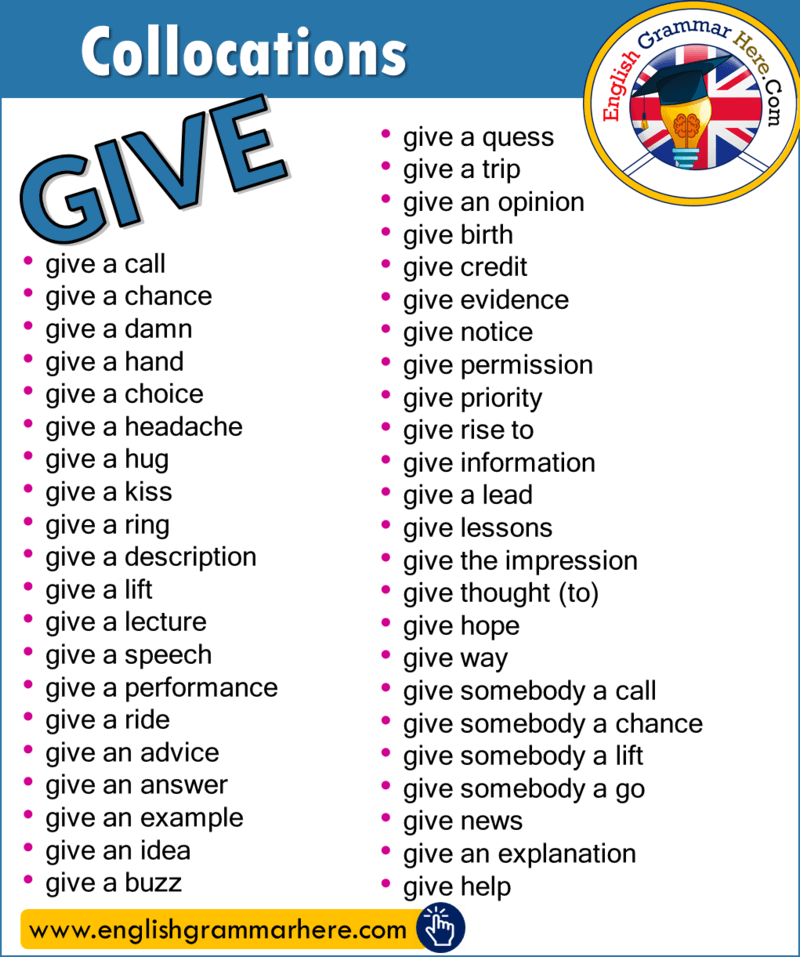Collocations with GIVE in English

English Phrases List, Collocations with GIVE in EnglishIf you want to look at Common Collocations List;

• give a call
• give a chance
• give a damn
• give a hand
• give a choice
• give a hug
• give a kiss
• give a ring
• give a description
• give a lift
• give a lecture
• give a speech
• give a performance
• give a ride
• give an example
• give an idea
• give a buzz

• give a quess
• give a trip
• give an opinion
• give birth
• give credit
• give evidence
• give notice
• give permission
• give priority
• give rise to
• give information